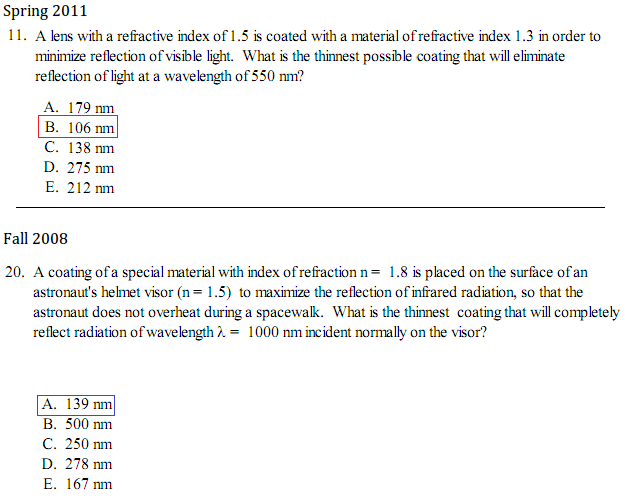# Thin-Film Interference: Coating a Lens - Answers found, explanation needed

• FishStik

#### FishStik

Thin-Film Interference: Coating a Lens -- Answers found, explanation needed!

## Homework StatementBoxed answers are the correct ones.

## Homework Equations

2L=(m+1/2)(λ/n) for constructive interference (maximum reflection, bright film)
2L=(m)(λ/n) for destructive interference (minimum reflection, dark film)

Where:
L = thickness of film
m = fringe count (for m=0,1,2,3,4...)
λ = wavelength of light
n = refractive index of coating

## The Attempt at a Solution

I got the correct answer (a) for Fall2008 #20 by using the equation for constructive interference, plugging in 0 for m, 1000 nm for λ, and 1.8 for n.
Now for Sp2011 #11, I took it as essentially the same question except this one is asking for minimum reflection, so I should use the equation for destructive interference. Doing that gets me (e), which is incorrect. The correct answer is (b), which is half of (e), implying that I should use the equation for constructive interference.

Am I missing a fundamental difference between the two problems besides one asking for minimum and another asking for maximum reflection? Thanks for the help.

Last edited:

The λ of the light in the coating is 550/n. The light must travel a distance of λ/2 in the coating to emerge from the coating out of phase with the reflected light byλ/2 and cause destructive interference. In the first example this means that the coating needs to be λ/4 thick.(106nm)
In the second example the logic is exactly the same but this time a phase change of λ/2 occurs at the junction of the coating and the visor of the helmet. A phase change of λ/2 occurs when there is reflection from a boundary when the light passes from high refractive index (1.8) into low refractive index (1.5). The wavelength of the light in the coating is 1000/n
A phase change of 0.5 of this wavelength occurs at the coating/visor junction. To MAXIMISE the reflection the 2 waves should emerge in phase therefore the path difference through the coating (2 x thickness) needs to be λ/2 giving a total phase difference of 1λ
In the first example there is no λ/2 phase difference at the junction between the coating and the lens because the refractive index of the lens is greater than the refractive index of the coating.

In the second example the logic is exactly the same but this time a phase change of λ/2 occurs at the junction of the coating and the visor of the helmet. A phase change of λ/2 occurs when there is reflection from a boundary when the light passes from high refractive index (1.8) into low refractive index (1.5).

The opposite is true: There is no phase change when the light reflects from a lower-index medium, and the phase of the reflected wave changes by pi when the light enters from a lower index medium to the boundary with a higher index one.

The pi phase change corresponds to half-wavelength optical path difference.

ehild

ehild... you are correct, I have checked my notes and got it the wrong way round!
I think that in the first question there is a phase change of pi at both surfaces but in the second question there is only 1 phase change of pi at the air/coating surface.
I think it makes no difference to the answers but what I wrote was confusing.
Apologies to FishStik and to you... and thanks.

You are welcomeehild